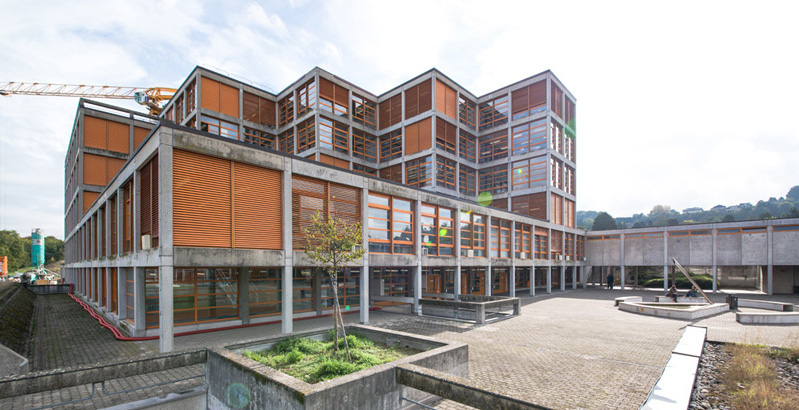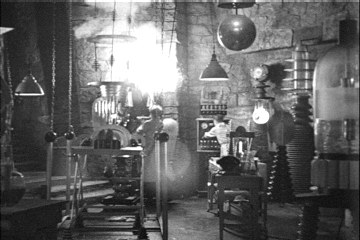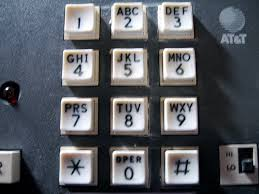# Programmation appliquée en ScalaCopyright © Cay S. Horstmann 2015# Higher-Order Functions

• In Scala, functions are values:
`val triple = (x: Int) => 3 * x`
• The type of `triple` is `Int => Int`
• You can pass `triple` to another function/method:
```1.to(10).map(triple)
// yields 3 6 9 12 ... 30```
• `map` is a higher-order function—it consumes a function
• Another useful higher-order function is `filter`. It consumes a function `T => Boolean`
```1.to(10).filter((x: Int) => x % 2 == 0)
// yields 2 4 6 8 10```
• Note that there was no need to store the function in a variable
• We will soon see other higher-order functions that produce functions

# Parameter Type Inference

• When you define a function, you normally need to specify the parameter types:
`val triple = (x : Int) => 3 * x`
• The return type is inferred. The type is `Int => Int`
• The parameter type can often be inferred as well:
```1.to(10).map((x) => 3 * x)
```
• Since `1.to(10)` is a `Seq[Int]`, the type of `x` is inferred to be `Int`
• Bonus: You can drop the `()`
`1.to(10).map(x => 3 * x)`
• Super bonus: Since `x` occurs exactly once, can drop `x =>` and use `_`:
`1.to(10).map(3 * _)`

# `reduceLeft`

• `s.reduceLeft(f)` computes `f(...f(f(s(0), s(1)), s(2))..., s(n-1))`
• Note that `f` is a function with two arguments
• `1.to(100).reduceLeft(_ + _)` is 1 + 2 + 3 + 4 + ... + 100 = 5050
• `s.reduceLeft((a, b) => if (a > b) a else b)` computes the maximum

# What is the Value Of ...

```1.to(100).map(_.toString).reduceLeft(_ + _)
```
1. The string `"5050"`
2. The string `"1 2 3 ... 98 99 100"`
3. A string of length 192
4. Something else (syntax error, other value, etc.)

# `flatMap`

• Given integer sequence `s`, easy to form all pairs (x, 0):
```s.map(x => (x, 0))
// Vector((1, 0), (2, 0), (3, 0), (4, 0))```
• What if we want to have all pairs (x, y) where x, y are elements of s?
• Try it by calling `map` twice:
```s.map(y => s.map(x => (x, y)))
// Vector(Vector((1,1), (2,1), (3,1), (4,1)),
//        Vector((1,2), (2,2), (3,2), (4,2)),
//        Vector((1,3), (2,3), (3,3), (4,3)),
//        Vector((1,4), (2,4), (3,4), (4,4)))```
• Close, but not quite. To “flatten out” the result, use `flatMap` instead:
```s.flatMap(y => s.map(x => (x, y)))
// Vector((1, 1), (1, 2), (1, 3), (1, 4), (2, 1), (2, 2), ..., (4, 4)) ```
• # Capturing the Enclosing Environment

• Consider this function:
```val n = 3
val fun = (x : Int) => n * x
// What is fun(2)?```
• `n` is not defined in the scope of `fun`, but that is ok. In the body of a function, you can use any variable from the enclosing scope.
• Doesn't seem a big deal—`n` is immutable, so it will always be 3. But consider this:
`def mulBy(n : Int) = (x : Int) => n * x`
• Huh? Let's call it:
`val quadruple = mulBy(4) // the function (x : Int) => 4 * x`
• And let's call that:
`quadruple(5) // yields 20`
• Each call to `mulBy` yields a different function.
• Each function has a different value of `n`
• Closure = function + binding of its free variables (i.e the variables that are not defined locally in the function)

# Where Did We Already Use Closures?

1. In the `reduceLeft` slide
2. In the `flatMap` slide
3. In both
4. In neither

# Lab1. You work with a buddy
2. One of you (the coder) writes the code, the other (the scribe) types up answers
3. When you get stuck, ask your buddy first!
4. Switch coder/scribe roles each lab
5. The coder submits the worksheet. Include the scribe's name in the worksheet!
6. The scribe submits answers. Include the coder's name in the report!

# Part 1: Life without Loops

1. Make a worksheet `Day3`. Type in
`val zones = java.util.TimeZone.getAvailableIDs`
. What do you get?
2. We want to get rid of the continents. Try this:
```zones.map(s => s.split("/"))
```
3. Ok, halfway there. Add a map that takes an array and yields `a(1)`. What did you do? What happens?
4. Hmmm, that's weird. There seem to be arrays of length < 2. How can you find them?
5. Ok, now get rid of them and try again. What did you do?
6. That's a lot of values. Can we get every tenth of them? (Hint: `grouped(10)`)

# Part 2: Reductions

1. Evaluate
`1.to(10).reduceLeft(_ * _)`
What do you get?
2. Write a function that computes n! in this way.
3. Surely you have written a factorial function before. How did you used to do it? Which approach do you like better?
4. Now we'd like to compute 2n with the same trick. How can you get a sequence of `n` copies of the number 2? Hint: `map`.
5. What is your function that computes 2n?
6. Given a `Seq[String]`, how can you use `reduceLeft` to concatenate them with spaces in between? Write a function `catSpace` that does this. For example, `catSpace(Vector("Mary", "had", "a", "lamb"))` should give a string `"Mary had a lamb"`
7. What happens when you try `1.to(10).reduceLeft(_ < _)`? Why?

# Part 3: Fun with `flatMap` and `reduceLeft`Martin Odersky, the inventor of Scala (as well as the charming husband of one of your instructors) likes to show off the power of Scala with a solution of the “phone mnemonics” problem. Given a phone number, what word or word sequence spells it on a touch-tone pad? For example, given the phone number 435 569 6753, one should come up with the sequence `HELLO WORLD` (and maybe others).

We can do better than what he did in his presentations. Over the next three labs, we'll solve this problem with our very restricted subset of Scala.

1. What interesting word can you make from the number 72252?
2. Declare a map that maps `'2'` to `"ABC"`, `'3'` to `"DEF"`, and so on. How do you do that? Pay attention that the keys should be characters and the values should be strings.
3. Actually, we want '2' to map to a `Vector` with three elements, `"A"`, `"B"`, `"C"`. But we don't want to type all that by hand. Transform your map by adding
```.map(e => (e._1, e._2.map(c => c.toString)))
```
Call the result `letters`—we'll need it in the next step.
4. Now it's trivial to find out the words that one can make with one digit. It's just `letters(d)`. Let's move on to two digits. Write a function that given two digits produces the set of all words (whether or not they make sense) that you can make with both.
`val wordsFor = (a: Char, b: Char) => ...`
For example, `wordsFor('5', '9')` contains "JW", "JX", "JY", "KW", "KX", "KY", "LW", "LX", "LY". Hint: `flatMap`